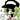# The Little Schemer 知识点整理（三）：用 cons 构筑恢宏

2018 年 12 月 05 日

## 自定义函数

### (rember a lat)

``````(define rember
(lambda (a lat)
(cond
((null? lat) (quote ()))
((eq? (car lat) a) (cdr lat))
(else (cons (car lat)
(rember a (cdr lat)))))))``````

### (firsts l)

``````(define firsts
(lambda (l)
(cond
((null? l) (quote ()))
(else (cons (car (car l))
(firsts (cdr l)))))))``````

### (insertR new old lat)

``````(define insertR
(lambda (new old lat)
(cond
((null? lat) (quote ()))
((eq? (car lat) old)
(cons old
(cons new (cdr lat))))
(else (cons (car lat)
(insertR new old (cdr lat)))))))``````

### (insertL new old lat)

``````(define insertL
(lambda (new old lat)
(cond
((null? lat) (quote ()))
((eq? (car lat) old) (cons new lat))
(else (cons (car lat)
(insertL new old (cdr lat)))))))``````

### (subst new old lat)

``````(define subst
(lambda (new old lat)
(cond
((null? lat) (quote ()))
((eq? (car lat) old) (cons new (cdr lat)))
(else (cons (car lat)
(subst new old (cdr lat)))))))``````

### (subst2 new o1 o2 lat)

``````(define subst2
(lambda (new o1 o2 lat)
(cond
((null? lat) (quote ()))
((or (eq? (car lat) o1) (eq? (car lat) o2))
(cons new (cdr lat)))
(else (cons (car lat)
(subst2 new o1 o2 (cdr lat)))))))``````

### (multirember a lat)

``````(define multirember
(lambda (a lat)
(cond
((null? lat) (quote ()))
((eq? (car lat) a) (multirember a (cdr lat)))
(else (cons (car lat)
(multirember a (cdr lat)))))))``````

### (multiinsertR new old lat)

``````(define multiinsertR
(lambda (new old lat)
(cond
((null? lat) (quote ()))
((eq? (car lat) old)
(cons old
(cons new
(multiinsertR new old (cdr lat)))))
(else (cons (car lat)
(multiinsertR new old (cdr lat)))))))``````

### (multiinsertL new old lat)

``````(define multiinsertL
(lambda (new old lat)
(cond
((null? lat) (quote ()))
((eq? (car lat) old)
(cons new
(cons old
(multiinsertL new old (cdr lat)))))
(else (cons (car lat)
(multiinsertL new old (cdr lat)))))))``````

### (multisubst new old lat)

``````(define multisubst
(lambda (new old lat)
(cond
((null? lat) (quote ()))
((eq? (car lat) old)
(cons new
(multisubst new old (cdr lat))))
(else (cons (car lat)
(multisubst new old (cdr lat)))))))``````

EOFTwinkle 的博客

© 2020